srdanova math

Recommended Posts

questions

1.Z÷(10^n)=?,Z-integers

2.write in abbreviated form (if the function can be final and natural)

2+5=7 , 2+10=12 , 2+15=17, 2+20=22 , 2+25=27 , 2+30=32 , 2+35=37 , 2+38=40,

2+40=42, 2+41=43 , 2+44=46 , 2+45=47, 2+47=49 , 2+50=52 ,2+57=59 , 2+60=62 ,

2+64=66, 2+70=72, 2+71=73 , 2+78=80 , 2+80=82 , 2+85=87 , 2+90=92 ,2+92=94

3.how to solve this current knowledge of mathematics:

along a (20m) ,deleted between 10 m and 15 m (b=5m) , wet get c (image)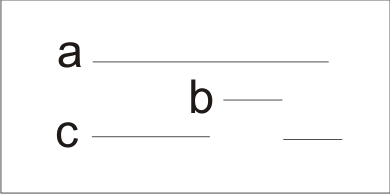Can mathematics explain the only two axiom that the rest are just evidence (experiments), if you think so join me show you Srdanova math, see you

__________________________________________________ ______________

I figure this way, from education school has 12 years, I was always the subject of mathematics and physics had the best grades, math deal amateur, studying mathematics I came to know that mathematics can be simplified and be connected (to be explained only with two axiom) and extend the mathematics that can solve math problems that present no solution.Marjanovic Srdan

M.Biljanica

natural axiom

What is " nature along "?

-nature along in figure 1

What is "point"?

-start (end) natural long in figure 2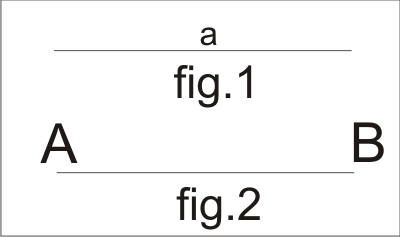What is the " basic rule "?

-basic rule is determined that the two ( more) longer only have to connect the points

[sn]-mathematical facts

[s1]-nature along

[s2]-point (natural meaning of)

Definition[natural along]-two points , distance between two points

CM (current mathematics)-[s1]-does not know , [s2]-point is not defined , so anything and everything

Share on other sites

As far as I can tell that makes no sense at all.

Share on other sites

NATURAL MATHEMATICS

Presupposition-natural long merge points in the direction of the first natural along AB

Process:

P1-AB..CD..ABC(AC)

to read- natural along AB to point B, is connected to the natural long CD to point C, shall be

read- along the ABC(AC) to point C , connecting with the natural long DE to point D is done

renaming of points , we get along ABCD(AD)

...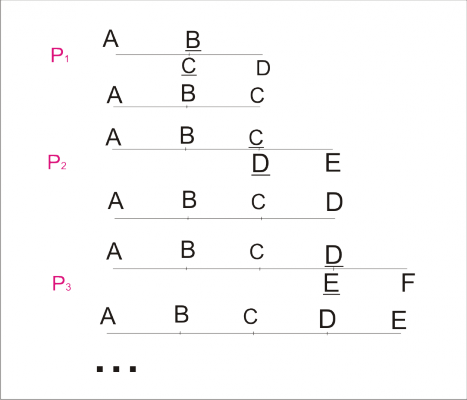[s3]-along (natural basis)

Definition[along]-the first and last point and the distance between points

CM-[s3]-does not know

_________________________________________________________________________________

Presupposition - All points of a longer (the infinite form) can be replaced with labels: (0), (0,1 ),...,

(0,1,2,3,4,5,6,7,8,9 ),...

Process:

P1-N (0) = {0,00,000,0000,...}

P2-N (0,1) = {0,1,10,11,100,...}

...

P10-N (0,1,2,3,4,5,6,7,8,9) = {0,1,2,3,4,5,6,7,8,9,10,11, ...}

...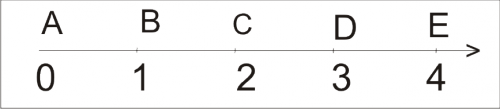[s4]-number along

[s5]-set of natural numbers N

We will use N (0,1,2,3,4,5,6,7,8,9) = {0,1,2,3,4,5,6,7,8,9,10,11,12,...}

Definition[number along]- a starting point (0), the last point at infinity

[number N]-The number 0 is the point 0

-Other numbers are longer, the first item is 0, the last point is the point of the name (number)

CM-[s4].does not know , [s5]-axiom

_______________________

Presupposition-Numbers have their points

Process: P1 0=(.0) P2 1={(.0),(.1)} P3 2={(.0),(.1),(.2)} P4 3={(.0),(.1),(.2),(.3)} P5 4={(.0),(.1),(.2),(.3),(.4)}...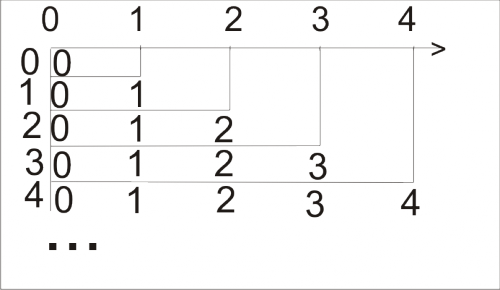[s6]-number points CM-[s6]does not know

Share on other sites

As far as I can tell that makes no sense at all.

A quick google search reveals that he spams this stuff all over the internet. He never really seems to answer anyone in a fashion that even remotely follows the question asked of him, so he is either a bot or doesn't know enough English to hold a conversation.

kumarevo: Please write out some prose an explain what it is you are talking about. Based on your post history throughout the internet you regularly fail Turing tests. Please show that you have some intent here other than using this site as a billboard on which to post your math-spam. We are a discussion forum, not a free publishing house.

Share on other sites

kumarevo: Please write out some prose an explain what it is you are talking about. Based on your post history throughout the internet you regularly fail Turing tests. Please show that you have some intent here other than using this site as a billboard on which to post your math-spam. We are a discussion forum, not a free publishing house.

I initially gave you three questions, first can be solved by the present knowledge of mathematics (so please solve it, you'll get an interesting result, which calls into question some sets of numbers), the second task will not be resolved because it knows that the current math functions can be final and only the set of natural numbers, the third task will not be resolved because the math does not know the current gap number and several forms of calculation subtraction.

what I've realized that the whole thing in math geometry, and that everything is a ratio of two (more) geometrical object in a line, plane, n-volume, I am totally rejected the historical legacy that has a current math, I started from the first geometry object (along natural) and the concept of point and a basic rule to connect the two (more) along the natural area this is the first axiom and it does not prove,each new object (concept, calculation, ...) occurs along the natural object or arising out along natural ...

Share on other sites

Presupposition-numbers have opposite points

Process:

P1 0=(s.0)

P2 1={(s.0),(s.1)}

P3 2={(s.0),(s.1),(s.2)}

P4 3={(s.0),(s.1),(s.2),(s.3)}

P5 4={(s.0),(s.1),(s.2),(s.3),(s.4)}

...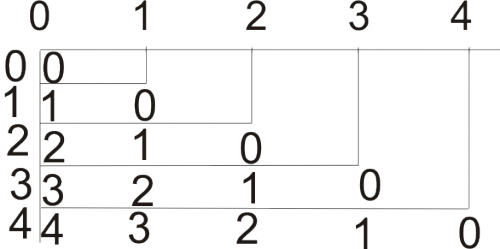...

[s7]-number opposite points

CM-[s7]does not know

______________

Presupposition-numbers are comparable with each other

Process:

P1-two numbers (a, b ) are comparable with each other - a> b, a =b, a <b, ).(=(>,=,<)

P2-three numbers (a, b, c) are comparable with each other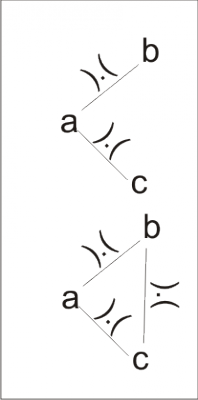P3-four numbers (a, b, c, d) are comparable with each other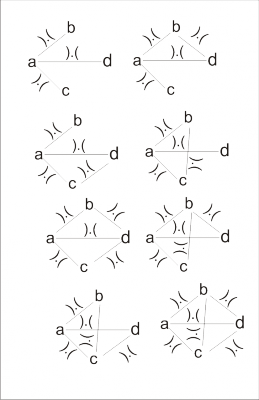...

[s8]-comparability numbers

CM-[s8]known two of comparability, comparability of three numbers(a number comparable with the numbers b and c),

comparability of the other knows.

Share on other sites

This is pointless.

Either start off by explaining carefully what you are talking about.

For example there is no use in saying "1.Z÷(10^n)=?,Z-integers" without saying what Z is and what n is.

Do the first three characters mean multiply Z by one (which is pointless) or are you numbering the example as being the first of 3.

Does "Z-integers" mean subtract the integers from Z?

We might be able to do that if you said what Z was.

So, if you can sort that out we might understand the first line of the nonsense you have written.

Once you have done that for all the stuff you have posted you might get a reply that moves us on.

Share on other sites

This is pointless.

Either start off by explaining carefully what you are talking about.

For example there is no use in saying "1.Z÷(10^n)=?,Z-integers" without saying what Z is and what n is.

Do the first three characters mean multiply Z by one (which is pointless) or are you numbering the example as being the first of 3.

Does "Z-integers" mean subtract the integers from Z?

We might be able to do that if you said what Z was.

So, if you can sort that out we might understand the first line of the nonsense you have written.

Once you have done that for all the stuff you have posted you might get a reply that moves us on.

$\frac{Z}{{{{10}^n}}}= {\rm ?}$

1.Z÷(10^n)=?

a={0,1,2,3,4,5,6,7,8,9} , b={1,2,3,4,5,6,7,8,9}

n=1 , Z÷10={...,(-2÷10),(-1÷10),(0÷10),(1÷10),(2÷10),...}={...,-0.2,-0.1,0,0.1,0.2,...}={Z,Z.b}

n=2 , Z÷100={Z,Z.b,Z.ab}

n=3 , Z÷1000={Z,Z.b,Zab,Zaab}

n=4 , Z÷10000={Z,Z.b,Z.ab,Zaab,Zaaab}

...

Share on other sites

!

Moderator Note

kumarevo,

Please respond to the questions being asked of you with clear and concise answers rather than restating things you've already said. This is a discussion forum, not a soapbox platform.

Create an account

Register a new account